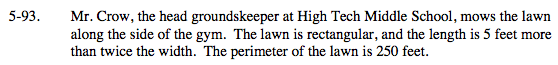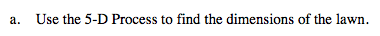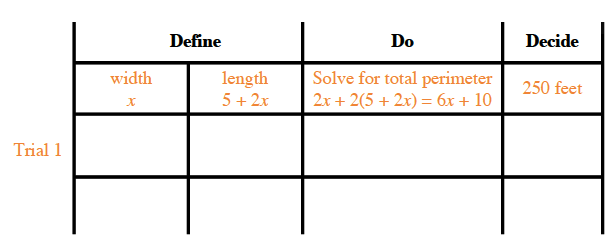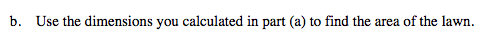### Home > MC2 > Chapter 5 > Lesson 5.3.1 > Problem5-93

5-93.
1. Mr. Crow, the head groundskeeper at High Tech Middle School, mows the lawn along the side of the gym. The lawn is rectangular, and the length is 5 feet more than twice the width. The perimeter of the lawn is 250 feet. Homework Help ✎

1. Use the 5-D Process to find the dimensions of the lawn.

2. Use the dimensions you calculated in part (a) to find the area of the lawn.Define the dimensions of the lawn based on the information that is given and create an expression to solve for the perimeter.

Now try values for x and run trials to see if they correspond to the given perimeter.The area of a rectangle is length • width.

The area of the lawn is 3,400 square feet.
Do you know how to get this answer?